# Cablecar

Funicular on Petrin (Prague) was 408 meters long and overcomes the difference 106 meters in altitude. Calculate the angle of climb.

x =  15.0584 °

### Step-by-step explanation:

$x=\frac{180\mathrm{°}}{\pi }\cdot \mathrm{arcsin}\left(106\mathrm{/}408\right)=15.0584\text{°}=15\mathrm{°}{3}^{\mathrm{\prime }}30\mathrm{"}$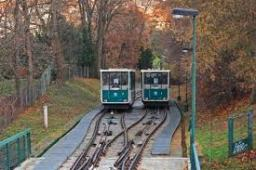Did you find an error or inaccuracy? Feel free to write us. Thank you!Tips to related online calculators

#### You need to know the following knowledge to solve this word math problem:

We encourage you to watch this tutorial video on this math problem:

## Related math problems and questions:Between cities A and B is route 13 km long of stúpanie average 7‰. Calculate the height difference of cities A and B.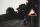A 5 km long road begins at an altitude 500 meters above sea level and ends at an altitude of 521 ASL. How many permille road rises?
• Altitude difference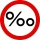What a climb in per mille of the hill long 4 km and the altitude difference is 6 meters?
• Climb in percentageThe height difference between points A and B is 475 m. Calculate the percentage of route climbing if the horizontal distance places A, B is 7.4 km.
• Height differenceWhat height difference is overcome if we pass a road 1 km long with a pitch21 per mille?
• RailwayRailway line had on 5.8 km segment climb 9 permille. How many meters track ascent?
• Slope of trackCalculate the average slope (in permille and even in degrees) of the rail tracks between Prievidza (309 m AMSL) and Nitrianske Pravno (354 m AMSL), if the track is 11 km long.
• The cable carThe cable car has a length of 3,5 kilometers and an angle of climb of 30 degrees. What is the altitude difference between the Upper and Lower stations?
• Railway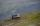Between points A, B, whose horizontal distance is 1.5 km railway line has 8promile climb. Between points B, C with horizontal distance of 900 m is climb 14promile. Calculate differences of altitudes between points A and C.
• Mountain railway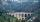Height difference between points A, B of railway line is 38.5 meters, their horizontal distance is 3.5 km. Determine average climb in permille up the track.
• Altitude angle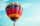In complete winds-free weather, the balloon took off and remained standing exactly above the place from which it took off. It is 250 meters away from us. How high did the balloon fly when we see it at an altitude angle of 25°?
• SSA and geometryThe distance between the points P and Q was 356 m measured in the terrain. The PQ line can be seen from the viewer at a viewing angle of 107° 22 '. The observer's distance from P is 271 m. Determine the viewing angle of P and observer.
• Map - climb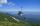On the map of High Tatras in scale 1:11000 are cable car stations in the Tatranska Lomnica and in the Skalnate Pleso with distance 354.6 mm. Altitude of this stations are 949 m and 1760 m. What is average angle of climb of this cable car track?
• Cable carCable car rises at an angle 45° and connects the upper and lower station with an altitude difference of 744 m. How long is "endless" tow rope?
• What percentageWhat percentage of the Earth’s surface is seen by an astronaut from a height of h = 350 km. Take the Earth as a sphere with the radius R = 6370 km
• KitesBoys run kite on a cable of 68 meters long. What is the kite altitude, if the angle from the horizontal plane is 72°?
• RailwaysRailways climb 7.4 ‰. Calculate the height difference between two points on the railway distant 3539 meters.